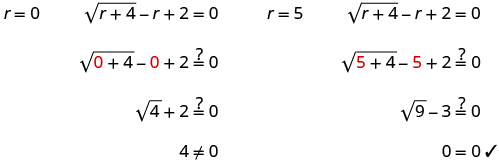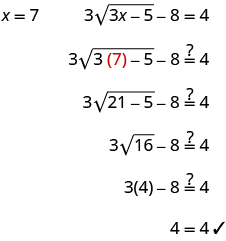# 9.6 Solve equations with square roots  (Page 2/6)

 Page 2 / 6

Solve: $\sqrt{x-2}+2=x$ .

$2,3$

Solve: $\sqrt{y-5}+5=y$ .

$5,6$

Solve: $\sqrt{r+4}-r+2=0$ .

## Solution

 $\sqrt{r+4}-r+2=0$ Isolate the radical. $\phantom{\rule{3.17em}{0ex}}\sqrt{r+4}=r-2$ Square both sides of the equation. $\phantom{\rule{2.26em}{0ex}}{\left(\sqrt{r+4}\right)}^{2}={\left(r-2\right)}^{2}$ Solve the new equation. $\phantom{\rule{3.63em}{0ex}}r+4={r}^{2}-4r+4$ It is a quadratic equation, so get zero on one side. $\phantom{\rule{5.17em}{0ex}}0={r}^{2}-5r$ Factor the right side. $\phantom{\rule{5.17em}{0ex}}0=r\left(r-5\right)$ Use the zero product property. $\phantom{\rule{1.99em}{0ex}}0=r\phantom{\rule{1em}{0ex}}0=r-5$ Solve the equation. $\phantom{\rule{2.09em}{0ex}}r=0\phantom{\rule{1em}{0ex}}r=5$ Check the answer.The solution is $r=5$ . $r=0$ is an extraneous solution.

Solve: $\sqrt{m+9}-m+3=0$ .

$7$

Solve: $\sqrt{n+1}-n+1=0$ .

$3$

When there is a coefficient in front of the radical, we must square it, too.

Solve: $3\sqrt{3x-5}-8=4$ .

## Solution

 $3\sqrt{3x-5}-8=4$ Isolate the radical. $\phantom{\rule{1.62em}{0ex}}3\sqrt{3x-5}=12$ Square both sides of the equation. $\phantom{\rule{0.67em}{0ex}}{\left(3\sqrt{3x-5}\right)}^{2}={\left(12\right)}^{2}$ Simplify, then solve the new equation. $\phantom{\rule{1.47em}{0ex}}9\left(3x-5\right)=144$ Distribute. $\phantom{\rule{1.59em}{0ex}}27x-45=144$ Solve the equation. $\phantom{\rule{3.7em}{0ex}}27x=189$ $\phantom{\rule{4.76em}{0ex}}x=7$ Check the answer.The solution is $x=7$ .

Solve: $2\sqrt{4a+2}-16=16$ .

$\frac{127}{2}$

Solve: $3\sqrt{6b+3}-25=50$ .

$\frac{311}{3}$

Solve: $\sqrt{4z-3}=\sqrt{3z+2}$ .

## Solution

$\begin{array}{cccc}& & & \phantom{\rule{5em}{0ex}}\sqrt{4z-3}=\sqrt{3z+2}\hfill \\ \\ \\ \text{The radical terms are isolated.}\hfill & & & \phantom{\rule{5em}{0ex}}\sqrt{4z-3}=\sqrt{3z+2}\hfill \\ \\ \\ \text{Square both sides of the equation.}\hfill & & & \phantom{\rule{4.07em}{0ex}}{\left(\sqrt{4z-3}\right)}^{2}={\left(\sqrt{3z+2}\right)}^{2}\hfill \\ \\ \\ \text{Simplify, then solve the new equation.}\hfill & & & \begin{array}{c}\phantom{\rule{5.5em}{0ex}}4z-3=3z+2\hfill \\ \phantom{\rule{6em}{0ex}}z-3=2\hfill \\ \phantom{\rule{7.61em}{0ex}}z=5\hfill \end{array}\hfill \\ \\ \\ \text{Check the answer.}\hfill & & & \\ \text{We leave it to you to show that 5 checks!}\hfill & & & \phantom{\rule{4em}{0ex}}\text{The solution is}\phantom{\rule{0.2em}{0ex}}z=5.\hfill \end{array}$

Solve: $\sqrt{2x-5}=\sqrt{5x+3}$ .

no solution

Solve: $\sqrt{7y+1}=\sqrt{2y-5}$ .

no solution

Sometimes after squaring both sides of an equation, we still have a variable inside a radical. When that happens, we repeat Step 1 and Step 2 of our procedure. We isolate the radical and square both sides of the equation again.

Solve: $\sqrt{m}+1=\sqrt{m+9}$ .

## Solution

$\begin{array}{cccc}& & & \phantom{\rule{2.43em}{0ex}}\sqrt{m}+1=\sqrt{m+9}\hfill \\ \\ \\ \text{The radical on the right side is isolated. Square both sides.}\hfill & & & \phantom{\rule{1.3em}{0ex}}{\left(\sqrt{m}+1\right)}^{2}={\left(\sqrt{m+9}\right)}^{2}\hfill \\ \\ \\ \text{Simplify—be very careful as you multiply!}\hfill & & & \phantom{\rule{0.09em}{0ex}}m+2\sqrt{m}+1=m+9\hfill \\ \text{There is still a radical in the equation.}\hfill & & & \\ \text{So we must repeat the previous steps. Isolate the radical.}\hfill & & & \phantom{\rule{3.58em}{0ex}}2\sqrt{m}=8\hfill \\ \\ \\ \text{Square both sides.}\hfill & & & \phantom{\rule{2.43em}{0ex}}{\left(2\sqrt{m}\right)}^{2}={\left(8\right)}^{2}\hfill \\ \\ \\ \text{Simplify, then solve the new equation.}\hfill & & & \phantom{\rule{4.04em}{0ex}}4m=64\hfill \\ & & & \phantom{\rule{4.54em}{0ex}}m=16\hfill \\ \\ \\ \text{Check the answer.}\hfill & & & \\ \\ \\ \text{We leave it to you to show that}\phantom{\rule{0.2em}{0ex}}m=16\phantom{\rule{0.2em}{0ex}}\text{checks!}\hfill & & & \text{The solution is}\phantom{\rule{0.2em}{0ex}}m=16.\hfill \end{array}$

Solve: $\sqrt{x}+3=\sqrt{x+5}$ .

$\text{no solution}$

Solve: $\sqrt{m}+5=\sqrt{m+16}$ .

$\text{no solution}$

Solve: $\sqrt{q-2}+3=\sqrt{4q+1}$ .

## Solution

$\begin{array}{cccc}& & & \phantom{\rule{7.29em}{0ex}}\sqrt{q-2}+3=\sqrt{4q+1}\hfill \\ \\ \\ \begin{array}{c}\text{The radical on the right side is isolated.}\hfill \\ \text{Square both sides.}\hfill \end{array}\hfill & & & \phantom{\rule{6.34em}{0ex}}{\left(\sqrt{q-2}+3\right)}^{2}={\left(\sqrt{4q+1}\right)}^{2}\hfill \\ \\ \\ \text{Simplify.}\hfill & & & \phantom{\rule{3.57em}{0ex}}q-2+6\sqrt{q-2}+9=4q+1\hfill \\ \\ \\ \begin{array}{c}\text{There is still a radical in the equation. So}\hfill \\ \text{we must repeat the previous steps. Isolate}\hfill \\ \text{the radical.}\hfill \end{array}\hfill & & & \phantom{\rule{8.41em}{0ex}}6\sqrt{q-2}=3q-6\hfill \\ \\ \\ \text{Square both sides.}\hfill & & & \phantom{\rule{7.52em}{0ex}}{\left(6\sqrt{q-2}\right)}^{2}={\left(3q-6\right)}^{2}\hfill \\ \\ \\ \text{Simplify, then solve the new equation.}\hfill & & & \phantom{\rule{8.11em}{0ex}}36\left(q-2\right)=9{q}^{2}-36q+36\hfill \\ \\ \\ \text{Distribute.}\hfill & & & \phantom{\rule{7.88em}{0ex}}36q-72=9{q}^{2}-36q+36\hfill \\ \\ \\ \begin{array}{c}\text{It is a quadratic equation, so get zero on}\hfill \\ \text{one side.}\hfill \end{array}\hfill & & & \phantom{\rule{11.06em}{0ex}}0=9{q}^{2}-72q+108\hfill \\ \\ \\ \text{Factor the right side.}\hfill & & & \begin{array}{c}\phantom{\rule{11.06em}{0ex}}0=9\left({q}^{2}-8q+12\right)\hfill \\ \phantom{\rule{11.06em}{0ex}}0=9\left(q-6\right)\left(q-2\right)\hfill \end{array}\hfill \\ \\ \\ \text{Use the zero product property.}\hfill & & & \begin{array}{cccc}\phantom{\rule{4.05em}{0ex}}q-6=0\hfill & & & q-2=0\hfill \\ \phantom{\rule{5.65em}{0ex}}q=6\hfill & & & \phantom{\rule{1.65em}{0ex}}q=2\hfill \end{array}\hfill \\ \\ \\ \begin{array}{c}\text{The checks are left to you. (Both solutions}\hfill \\ \text{should work.)}\hfill \end{array}\hfill & & & \phantom{\rule{4em}{0ex}}\text{The solutions are}\phantom{\rule{0.2em}{0ex}}q=6\phantom{\rule{0.2em}{0ex}}\text{and}\phantom{\rule{0.2em}{0ex}}q=2.\hfill \end{array}$

Solve: $\sqrt{y-3}+2=\sqrt{4y+2}$ .

$\text{no solution}$

Solve: $\sqrt{n-4}+5=\sqrt{3n+3}$ .

$\text{no solution}$

## Use square roots in applications

As you progress through your college courses, you’ll encounter formulas that include square roots in many disciplines. We have already used formulas to solve geometry applications.

We will use our Problem Solving Strategy for Geometry Applications, with slight modifications, to give us a plan for solving applications with formulas from any discipline.

## Solve applications with formulas.

1. Read the problem and make sure all the words and ideas are understood. When appropriate, draw a figure and label it with the given information.
2. Identify what we are looking for.
3. Name what we are looking for by choosing a variable to represent it.
4. Translate into an equation by writing the appropriate formula or model for the situation. Substitute in the given information.
5. Solve the equation using good algebra techniques.
6. Check the answer in the problem and make sure it makes sense.
7. Answer the question with a complete sentence.

One-fourth of the candies in a bag of M&M’s are red. If there are 23 red candies, how many candies are in the bag?
rectangular field solutions
What is this?
Donna
the proudact of 3x^3-5×^2+3 and 2x^2+5x-4 in z7[x]/ is
?
Choli
a rock is thrown directly upward with an initial velocity of 96feet per second from a cliff 190 feet above a beach. The hight of tha rock above the beach after t second is given by the equation h=_16t^2+96t+190
Usman
Stella bought a dinette set on sale for $725. The original price was$1,299. To the nearest tenth of a percent, what was the rate of discount?
44.19%
Scott
40.22%
Terence
44.2%
Orlando
I don't know
Donna
if you want the discounted price subtract $725 from$1299. then divide the answer by $1299. you get 0.4419... but as percent you get 44.19... but to the nearest tenth... round .19 to .2 and you get 44.2% Orlando you could also just divide$725/$1299 and then subtract it from 1. then you get the same answer. Orlando p mulripied-5 and add 30 to it Tausif Reply p mulripied-5 and add30 Tausif p mulripied-5 and addto30 Tausif Can you explain further Monica Reply p mulripied-5 and add to 30 Tausif How do you find divisible numbers without a calculator? Jacob Reply TAKE OFF THE LAST DIGIT AND MULTIPLY IT 9. SUBTRACT IT THE DIGITS YOU HAVE LEFT. IF THE ANSWER DIVIDES BY 13(OR IS ZERO), THEN YOUR ORIGINAL NUMBER WILL ALSO DIVIDE BY 13!IS DIVISIBLE BY 13 BAINAMA When she graduates college, Linda will owe$43,000 in student loans. The interest rate on the federal loans is 4.5% and the rate on the private bank loans is 2%. The total interest she owes for one year was $1,585. What is the amount of each loan? Ariana Reply Sean took the bus from Seattle to Boise, a distance of 506 miles. If the trip took 7 2/3 hours, what was the speed of the bus? Kirisma Reply 66miles/hour snigdha How did you work it out? Esther s=mi/hr 2/3~0.67 s=506mi/7.67hr = ~66 mi/hr Orlando hello, I have algebra phobia. Subtracting negative numbers always seem to get me confused. Alicia Reply what do you need help in? Felix subtracting a negative....is adding!! Heather look at the numbers if they have different signs, it's like subtracting....but you keep the sign of the largest number... Felix for example.... -19 + 7.... different signs...subtract.... 12 keep the sign of the "largest" number 19 is bigger than 7.... 19 has the negative sign... Therefore, -12 is your answer... Felix —12 Niazmohammad Thanks Felix.l also get confused with signs. Esther Thank you for this Shatey ty Graham think about it like you lost$19 (-19), then found $7(+7). Totally you lost just$12 (-12)
Annushka
I used to struggle a lot with negative numbers and math in general what I typically do is look at it in terms of money I have -$5 in my account I then take out 5 more dollars how much do I have in my account well-$10 ... I also for a long time would draw it out on a number line to visualize it
Meg
practicing with smaller numbers to understand then working with larger numbers helps too and the song/rhyme same sign add and keep opposite signs subtract keep the sign of the bigger # then you'll be exact
Meg
Bruce drives his car for his job. The equation R=0.575m+42 models the relation between the amount in dollars, R, that he is reimbursed and the number of miles, m, he drives in one day. Find the amount Bruce is reimbursed on a day when he drives 220 miles
168.50=R
Heather
john is 5years older than wanjiru.the sum of their years is27years.what is the age of each
46
mustee
j 17 w 11
Joseph
john is 16. wanjiru is 11.
Felix
27-5=22 22÷2=11 11+5=16
Joyce
I don't see where the answers are.
Ed
Cindy and Richard leave their dorm in Charleston at the same time. Cindy rides her bicycle north at a speed of 18 miles per hour. Richard rides his bicycle south at a speed of 14 miles per hour. How long will it take them to be 96 miles apart?
3
Christopher
18t+14t=96 32t=96 32/96 3
Christopher
show that a^n-b^2n is divisible by a-bBy OpenStaxBy Brooke DelaneyBy Janet ForresterBy John GabrieliBy OpenStaxBy Donyea SweetsBy Laurence BailenBy David CoreyBy Anh DaoBy Marion Cabalfin